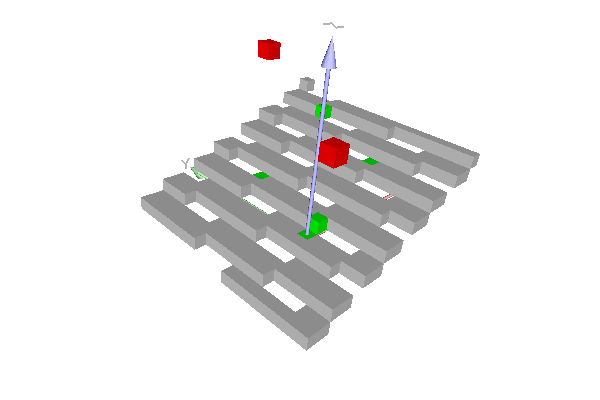DGtal  1.3.beta
io/viewers/viewer3D-7bis-planes.cpp

This example shows the recognition of a simple digital plane with the Chord algorithm (class DGtal::ChordNaivePlaneComputer). Green points belong to the naive plane. There is no naive plane containing the green points and any one of the red points.

Naive plane recognition (known axis) with Chord algorithm
\$ ./examples/io/viewers/viewer3D-7bis-planesPiece of naive plane containing the four green points. There is no naive plane containing also any one of the red points.
#include <cstdlib>
#include <iostream>
#include "DGtal/base/Common.h"
#include "DGtal/helpers/StdDefs.h"
#include "DGtal/geometry/surfaces/ChordNaivePlaneComputer.h"
#include "DGtal/io/viewers/Viewer3D.h"
using namespace std;
using namespace DGtal;
// Standard services - public :
template <typename Viewer3D, typename Domain, typename Predicate>
void
const Domain & domain, const Predicate & pred )
{
for ( typename Domain::ConstIterator itB = domain.begin(), itE = domain.end();
itB != itE; ++itB )
{
if ( pred( *itB ) )
viewer << *itB;
}
}
int main( int argc, char** argv )
{
using namespace Z3i;
QApplication application(argc,argv);
trace.beginBlock ( "Testing class ChordNaivePlaneComputer" );
unsigned int nbok = 0;
unsigned int nb = 0;
typedef PlaneComputer::Primitive Primitive;
PlaneComputer plane;
plane.init( 2, 1, 1 );
Point pt0( 0, 0, 0 );
bool pt0_inside = plane.extend( pt0 );
trace.info() << "(" << nbok << "/" << nb << ") Plane=" << plane
<< std::endl;
Point pt1( 8, 1, 3 );
bool pt1_inside = plane.extend( pt1 );
++nb; nbok += pt1_inside == true ? 1 : 0;
trace.info() << "(" << nbok << "/" << nb << ") add " << pt1
<< " Plane=" << plane << std::endl;
Point pt2( 2, 7, 1 );
bool pt2_inside = plane.extend( pt2 );
++nb; nbok += pt2_inside == true ? 1 : 0;
trace.info() << "(" << nbok << "/" << nb << ") add " << pt2
<< " Plane=" << plane << std::endl;
Point pt3( 0, 5, 12 );
bool pt3_inside = plane.extend( pt3 );
++nb; nbok += pt3_inside == false ? 1 : 0;
trace.info() << "(" << nbok << "/" << nb << ") add " << pt3
<< " Plane=" << plane << std::endl;
Point pt4( -5, -5, 10 );
bool pt4_inside = plane.extend( pt4 );
++nb; nbok += pt4_inside == false ? 1 : 0;
trace.info() << "(" << nbok << "/" << nb << ") add " << pt4
<< " Plane=" << plane << std::endl;
Point pt5 = pt0 + pt1 + pt2 + Point( 0, 0, 1 );
bool pt5_inside = plane.extend( pt5 );
++nb; nbok += pt5_inside == true ? 1 : 0;
trace.info() << "(" << nbok << "/" << nb << ") add " << pt5
<< " Plane=" << plane << std::endl;
Point pt6 = Point( 1, 0, 1 );
bool pt6_inside = plane.extend( pt6 );
++nb; nbok += pt6_inside == true ? 1 : 0;
trace.info() << "(" << nbok << "/" << nb << ") add " << pt5
<< " Plane=" << plane << std::endl;
Primitive strip = plane.primitive();
trace.info() << "strip=" << strip
<< " axis=" << strip.mainAxis()
<< " axiswidth=" << strip.axisWidth()
<< " diag=" << strip.mainDiagonal()
<< " diagwidth=" << strip.diagonalWidth()
<< std::endl;
++nb; nbok += strip.axisWidth() < 1.0 ? 1 : 0;
trace.info() << "(" << nbok << "/" << nb << ") axiswidth < 1 "
<< std::endl;
++nb; nbok += strip.diagonalWidth() < sqrt(3.0) ? 1 : 0;
trace.info() << "(" << nbok << "/" << nb << ") axiswidth < sqrt(3) "
<< std::endl;
trace.emphase() << ( nbok == nb ? "Passed." : "Error." ) << endl;
MyViewer viewer;
viewer.show();
Color red( 255, 0, 0 );
Color green( 0, 255, 0 );
Color grey( 200, 200, 200 );
Domain domain( Point( -5, -5, -5 ), Point( 12, 12, 12 ) );
viewer << ( pt0_inside ? CustomColors3D( green, green ) : CustomColors3D( red, red ) ) << pt0;
viewer << ( pt1_inside ? CustomColors3D( green, green ) : CustomColors3D( red, red ) ) << pt1;
viewer << ( pt2_inside ? CustomColors3D( green, green ) : CustomColors3D( red, red ) ) << pt2;
viewer << ( pt3_inside ? CustomColors3D( green, green ) : CustomColors3D( red, red ) ) << pt3;
viewer << ( pt4_inside ? CustomColors3D( green, green ) : CustomColors3D( red, red ) ) << pt4;
viewer << ( pt5_inside ? CustomColors3D( green, green ) : CustomColors3D( red, red ) ) << pt5;
viewer << ( pt6_inside ? CustomColors3D( green, green ) : CustomColors3D( red, red ) ) << pt6;
viewer << CustomColors3D( grey, grey );
displayPredicate( viewer, domain, plane );
return application.exec();
}
// //
DGtal::ChordNaivePlaneComputer
Aim: A class that contains the chord-based algorithm for recognizing pieces of digital planes of give...
Definition: ChordNaivePlaneComputer.h:157
DGtal::HyperRectDomain< Space >
DGtal::Trace::endBlock
double endBlock()
DGtal::Color
Structure representing an RGB triple with alpha component.
Definition: Color.h:66
DGtal::ChordNaivePlaneComputer::init
void init(Dimension axis, InternalScalar widthNumerator=NumberTraits< InternalScalar >::ONE, InternalScalar widthDenominator=NumberTraits< InternalScalar >::ONE)
DGtal::Trace::emphase
std::ostream & emphase()
DGtal::trace
Trace trace
Definition: Common.h:154
displayPredicate
void displayPredicate(Viewer3D &viewer, const Domain &domain, const Predicate &pred)
Definition: viewer3D-7-planes.cpp:64
DGtal::Display3D::updateDisplay
@ updateDisplay
Definition: Display3D.h:249
DGtal::Trace::beginBlock
void beginBlock(const std::string &keyword="")
DGtal::Trace::info
std::ostream & info()
DGtal::Viewer3D
Definition: Viewer3D.h:135
DGtal
DGtal is the top-level namespace which contains all DGtal functions and types.
DGtal::HyperRectDomain::end
const ConstIterator & end() const
Definition: HyperRectDomain.h:201
main
int main(int argc, char **argv)
Definition: testArithmeticDSS-benchmark.cpp:147
DGtal::HyperRectDomain_Iterator
Iterator for HyperRectDomain.
Definition: HyperRectDomain_Iterator.h:142
domain
Domain domain
Definition: testProjection.cpp:88
DGtal::Viewer3D::show
virtual void show()
Overload QWidget method in order to add a call to updateList() method (to ensure that the lists are w...
DGtal::HyperRectDomain::begin
const ConstIterator & begin() const
Definition: HyperRectDomain.h:176
Point
MyPointD Point
Definition: testClone2.cpp:383